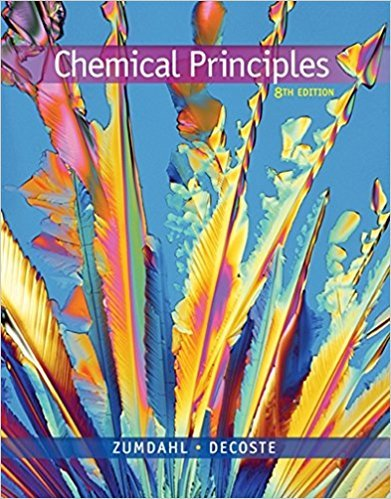×
Get Full Access to Chemical Principles - 8 Edition - Chapter 20 - Problem 20.22
Get Full Access to Chemical Principles - 8 Edition - Chapter 20 - Problem 20.22

×

# Solution: The curie (Ci) is a commonly used unit forISBN: 9781305581982 176

## Solution for problem 20.22 Chapter 20

Chemical Principles | 8th Edition

• Textbook Solutions
• 2901 Step-by-step solutions solved by professors and subject experts
• Get 24/7 help from StudySoup virtual teaching assistantsChemical Principles | 8th Edition

4 5 1 234 Reviews
27
2
Problem 20.22

The curie (Ci) is a commonly used unit for measuring nuclear radioactivity: 1 curie of radiation is equal to 3.7 3 1010 decay events per second (the number of decay events from 1 g of radium in 1 s). a. What mass of Na2 38SO4 has an activity of 10.0 mCi? Sulfur-38 has an atomic mass of 38.0 u and a halflife of 2.87 h. b. How long does it take for 99.99% of a sample of sulfur-38 to decay?

Step-by-Step Solution:
Step 1 of 3

CHEM 372 Week 2 Notes  Week 2: Day 1  ∆G < 0 exergonic, so ∆G > 0 Endergonic  Catabolism – breaking down molecules and releasing energy  Pathways o How to make sure you get one pathway and not the other pathway if you have both Cat./Ana.  Regulation is the trick to get the reaction where you want it to go o Regulation – activation/inhibiting enzymes to introduce/remove a barrier to a pathway  Glycolysis (downward)  Makes one reaction faster than another  Allosteric Inhibitors  Separate pathways – share begin/end points o Activate first step of desired reaction o Inhibit first step of reverse reaction o Ex: Fatty acid metabolism o Ex: common

Step 2 of 3

Step 3 of 3

##### ISBN: 9781305581982

Chemical Principles was written by and is associated to the ISBN: 9781305581982. This textbook survival guide was created for the textbook: Chemical Principles, edition: 8. This full solution covers the following key subjects: . This expansive textbook survival guide covers 20 chapters, and 2754 solutions. The answer to “The curie (Ci) is a commonly used unit for measuring nuclear radioactivity: 1 curie of radiation is equal to 3.7 3 1010 decay events per second (the number of decay events from 1 g of radium in 1 s). a. What mass of Na2 38SO4 has an activity of 10.0 mCi? Sulfur-38 has an atomic mass of 38.0 u and a halflife of 2.87 h. b. How long does it take for 99.99% of a sample of sulfur-38 to decay?” is broken down into a number of easy to follow steps, and 80 words. The full step-by-step solution to problem: 20.22 from chapter: 20 was answered by , our top Chemistry solution expert on 12/23/17, 04:53PM. Since the solution to 20.22 from 20 chapter was answered, more than 270 students have viewed the full step-by-step answer.

Unlock Textbook Solution